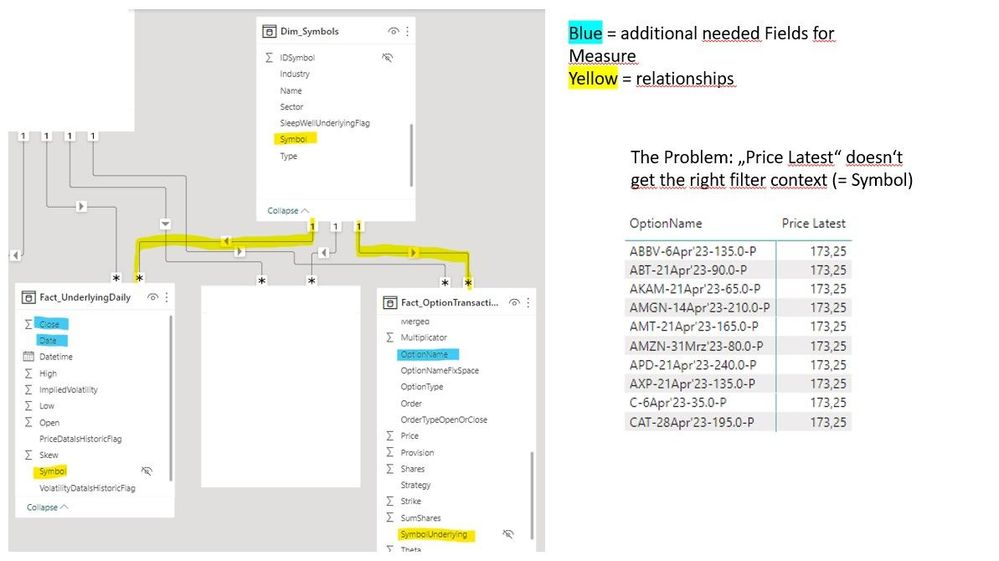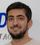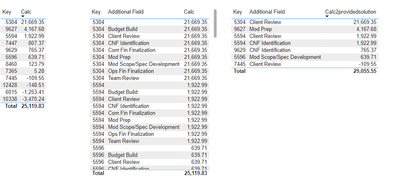cancel
Showing results for
Did you mean:Frequent Visitor

## Calculations on 2 fact Tables connected via dimension Table

Hi,

I need to calculate a latest price from a fact Table (UnderlyingDaily), but in my visual I need to place dimension fields from another fact Table. My current "Price Latest" measure only works if I use "Symbol" Field from "Dim_Symbol".

Is there a measure (e.g. with RELATED() function) that can solve my problem or do I need to adjust my relationships/tables?

Many Thanks, Fabian

``````Price Latest =
CALCULATE(
AVERAGE(Fact_UnderlyingDaily[Close]),
Fact_UnderlyingDaily[Date] = MAX(Fact_UnderlyingDaily[Date])
)``````

My data model setup:1 ACCEPTED SOLUTIONSolution Sage

Hi @fab_incher ,

Not sure, but Based on your explanation, I think you need the measure below:

``````Measure =
CALCULATE (
[Price Latest],
CROSSFILTER ( Fact_OptionTransaction[Symbol], Dim_Symbols[Symbol], BOTH )
)``````

If this answer solves your problem, give it a thumbs up and accept it as a solution so the others would find what they need easier.

Regards,

Loran

2 REPLIES 2New Member

Hi,

I have the same scenario, but my first table Fact_underlying_daily has symbols, for which there is no data into the second table. When I use the already provided solution, I only can calculate the values which are in the second fact table. Is there any possibility to have all the values from the first fact table and the additional fields from the second table. Currently when the calculation is correct, the field from the second fact table is visualized many times.Solution Sage

Hi @fab_incher ,

Not sure, but Based on your explanation, I think you need the measure below:

``````Measure =
CALCULATE (
[Price Latest],
CROSSFILTER ( Fact_OptionTransaction[Symbol], Dim_Symbols[Symbol], BOTH )
)``````

If this answer solves your problem, give it a thumbs up and accept it as a solution so the others would find what they need easier.

Regards,

Loran